Paluu  /  Back

25 week old R. tomentosum x R. minus (Hachmann) seed plants
R. tomentosum chromosome count tetraploid (2n = 52)
and minus
(Hachmann) diploid? (2n = 26?)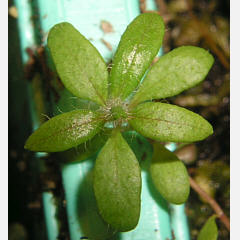tomentosum x minus 1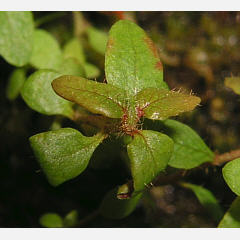tomentosum x minus 2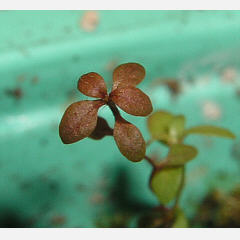tomentosum x minus 3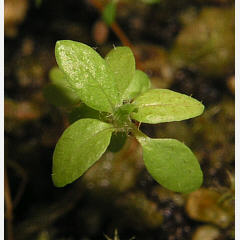tomentosum x minus 4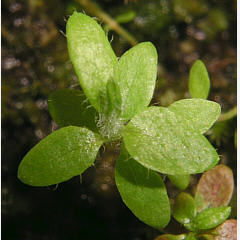tomentosum x minus 5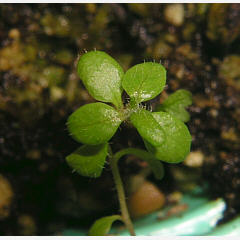tomentosum x minus 6

25 week old R. tomentosum x R. minus (Hachmann) seed plants
R. tomentosum chromosome count tetraploid (2n = 52)
and minus
(Hachmann) diploid? (2n = 26?)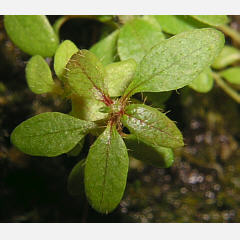tomentosum x minus 7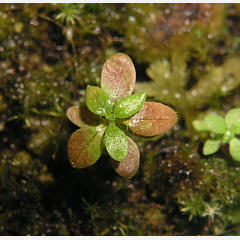tomentosum x minus 8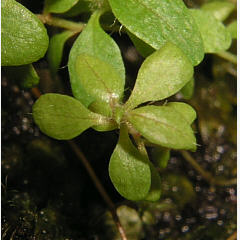tomentosum x minus 9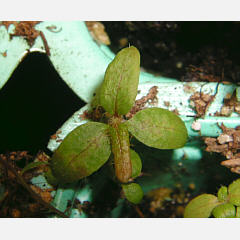tomentosum x minus 10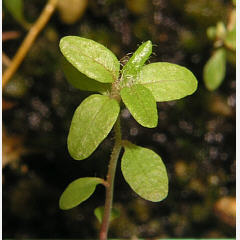tomentosum x minus 11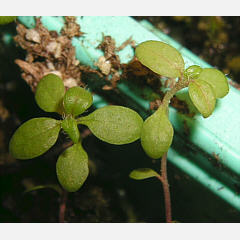tomentosum x minus 12